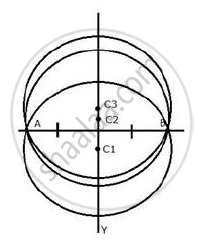# The locus of the centres of all circles passing through two fixed points. - Mathematics

Sum

Describe the locus for questions 1 to 13 given below:

The locus of the centres of all circles passing through two fixed points.

#### SolutionThe locus of the centre of all the circles which pass through two fixed points will be the perpendicular bisector of the line segment joining the two given fixed points.

Concept: Theorems Based on Loci
Is there an error in this question or solution?

#### APPEARS IN

Selina Concise Maths Class 10 ICSE
Chapter 16 Loci (Locus and Its Constructions)
Exercise 16 (B) | Q 9 | Page 241